## Average Function and Different Ways to Use It

In this lesson you can learn how to use Average function. The average function in Excel is a feature that would use the arguments to return with average of those arguments. However, it would only use the cells that are containing numbers to calculate the average. The advantage of this function is that it can enter addresses of individual cells and also the whole range. Average is also available in the status bar. Parameters (Syntax) The syntax of average has one obligatory argument, while others are optional.The syntax looks like this: =AVERAGE(number1; [number2];…).Number1: This is a required argument, and could be cell reference, a range that you would like to have the average of.

Number2: This is optional. It could also contain reference, range, and these ones reach maximum of 255.Take a look at the picture above.

Function AVERAGE doesn’t care about empty cells and text in the cell. In the first and third table function AVERAGE there is a different average than in the second table.

It is very easy to make a mistake. Remember about zeros in cells.

## Example 1 Average Between Two Values

{=AVERAGE(IF((A1:A10>=B1)*(A1:A10<=B2),A1:A10))}

B1 and B2 are the values between which you want to calculate average.

This is an array formula so accept it by CTRL + SHIFT + ENTER.

## Example 2 To avoid errors

Use this formula when you can haven’t got any data in your worksheet.

=IF(ISERROR(AVERAGE(A1:A5)),”No Data”,AVERAGE(A1:A5))

If your data in A1:A5 are missing, Excel will show “No data”. If it is ok with your data there will be average calculated.

## Example 3 Average of 10 largest values

{=AVERAGE(LARGE(A1:A50,ROW(1:10)))}

This formula gives you the average of 10 largest values in A1:A50 range as the result.

This is an array formula so accept it by CTRL + SHIFT + ENTER.

## Example 4 Average with some data missing

How to calculate values average in case of zeros or missing data. Use that formula:

{=AVERAGE(IF(\$A\$1:\$A\$5<>0,\$A\$1:\$A\$5))}

This is an array formula so accept it by CTRL + SHIFT + ENTER.This formula doesn’t count blanks and zeros. In this case average is (2+5+6+3):4. Formula doesn’t calculate value in A2 cell. There will be the same when A2 will be blank or text.

## Example 5: Simple Average Function Usage

For the past six months, we have been making sales, and we have decided to find out how much we have averagely made under those six months, so we could find a perfectly suitable ways of closing. This is because we want to reach a goal.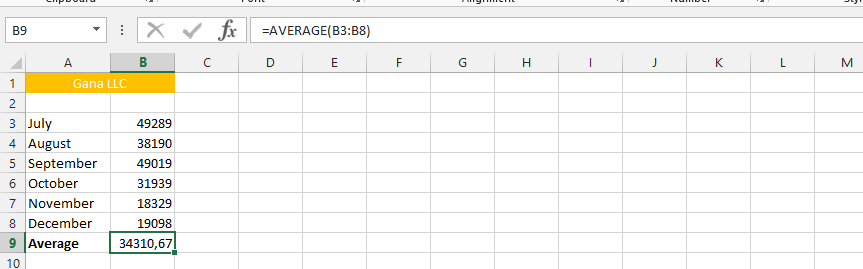## Example 6: Customized Average Usage

The same data as the previous one, but this time I am looking at a specific period. It becomes necessary to know when the average was highest, which helps the comprehension of company’s performance.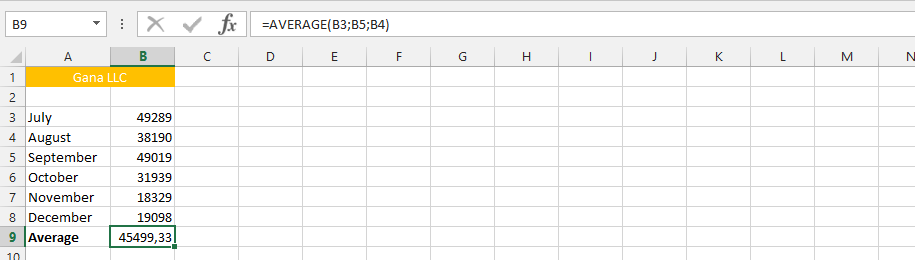## Example 7: Average Functions Multiplied

This is the example that allows us to multiply the average of both sales and products. However, this only works under a whole new scenario. It addresses the issues that the first column is the price, while the other column is the quantity of products we sold.## Example 8: AVERAGE Evaluation

We have our business going, but we would like to make sure that we are having the appropriate value, because it has been determined that at any given time, the average should be above the expenses. We are now going to use the average function, in consideration to evaluating the financial performance of the company on average level.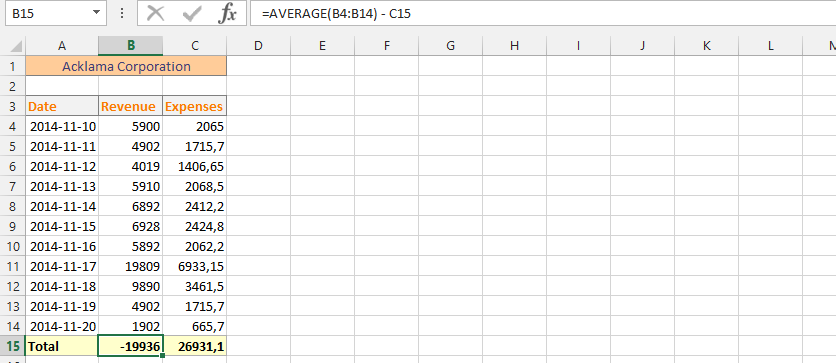## Example 9: Double Average Evaluation

This is the perfect example where as we are solely focusing on our average performance when making the evaluation. The business has the very same data as previous example. But, this time the situation is very different, because we are solely evaluating average on both columns.## Example 10: Average with Text

We have made a mistake of labelling all our data with text, and we really need to do the average of our sales.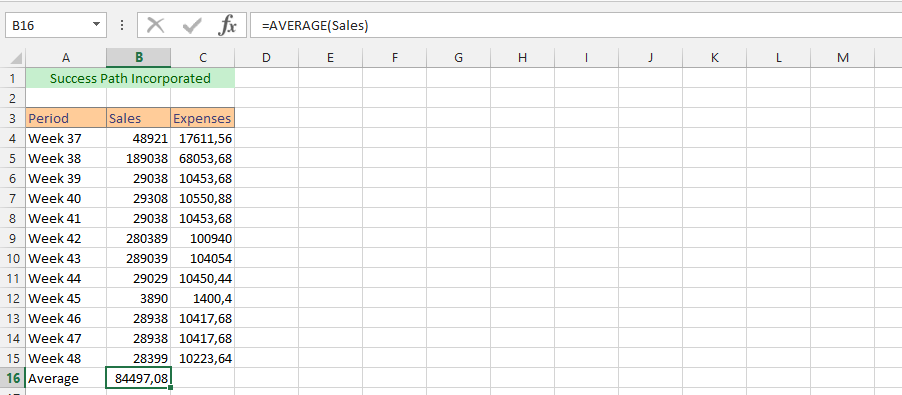## Example 11: Evaluating with Text

With the same situation as the previous one, it is necessary to use the text to find out the average financial performance.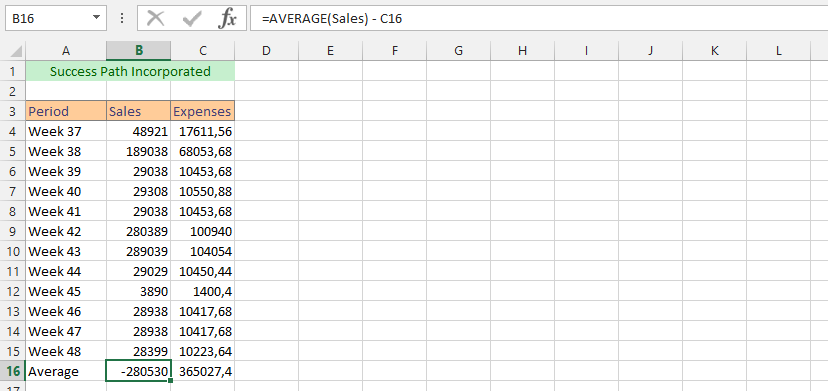## Example 12: Double Evaluation with Text

This is using the text to do another double evaluation. We are going to evaluate this in form of average.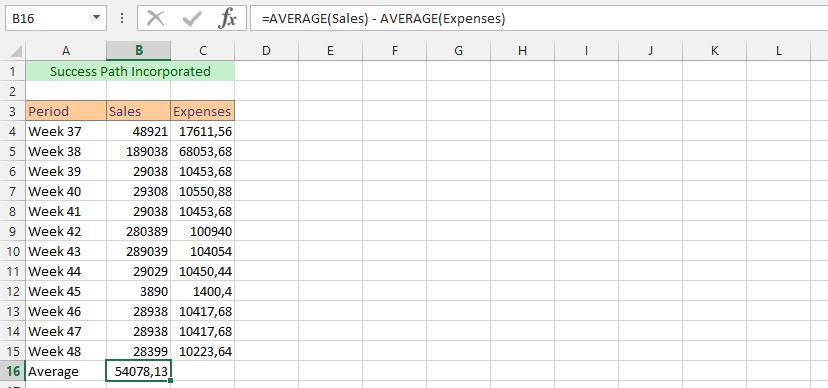Just as the previous example, but this time the information is in another spreadsheet.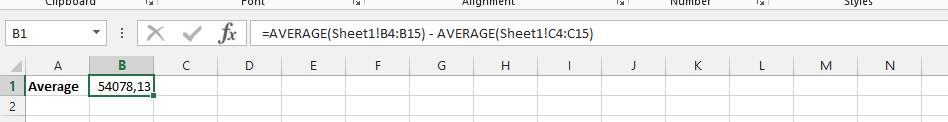## Example 14: Average from Multiple Files

The business is three years old, we have our data spread in three different documents, including our current file. Our business is now in need of knowledge, and we would like to know how the business is doing on an average level. We are therefore taking data from those other two files, in other to get the answer that we are looking for.## Template

```Further reading: